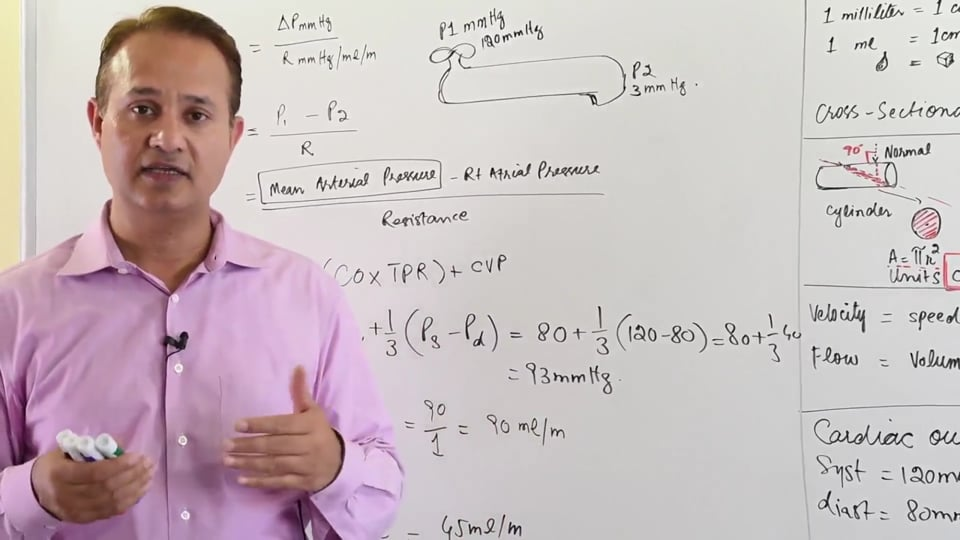# Blood Flow Hemodynamics

{{isShortDescriptionLonger && !showFullDescription ? shortDescription.slice(0, 250) : shortDescription}}... See {{showFullDescription? 'less' : 'more'}}

#### Write A New Comment

This video presents: Blood flow in the cardiovascular system. Factors affecting the blood flow. Method to calculate the blood flow.

PHYSIOLGY LECTURE # 4 STUDY NOTES:

HEMODYNAMICS - BLOOD FLOW

Basic Metric system:

1m = 39.37 inches

1ml = 1 cubic centimetre (cm^3)

A cubic centimetre is diagrammed as a small cube which has all its lengths equal to 1 centimetre.

Cross-Sectional Area

The cardiovascular system is seen as a network of cylindrical tubes. If a cylinder is cut at normal (right angle) and the cross section viewed, it will appear as a circle. The area of a circle is calculated by the formula, (). The radius of the circle is determined by measuring the length from the centre of circle to any point along its circumference. The unit for the cross sectional area is cm^2.

Velocity vs. Flow

Velocity is defined as the distance covered in unit time (cm/s). For simplicity, velocity is defined as the speed of movement of a particle. Velocity of a particle in a fluid is independent of the total volume of the fluid itself. The velocity of the blood is equal to the distance it covers in unit time, irrespective of the volume moved.

Flow however is dependant of the fluid volume. Flow refers to the volume of fluid that passes through a cross sectional area in unit time. With respect to hemodynamics, flow is measured as the volume of blood that passes through a particular area in unit time (ml/min).

Formulae:

➢ Velocity (cm/s) = Flow/Cross Sectional Area

= Q/A (Unit: [ml/m]/cm^2)

Assumptions: For the purpose of this lecture, the flow of blood in an average, healthy adult is assumed as 5L/min, which is also the cardiac output.

After being pumped from the heart, the blood first flows into the aorta and then into the major arteries. From there, the blood enters arterioles of the respective functional organ systems. The arterioles divide into small capillaries which anastomose with capillaries of the venules within the organ system. The venules empty into veins and the veins after collecting blood from all parts of the body, empty into the Superior and Inferior Vena Cava which deliver the blood back to the heart, completing the circuit. Together these components of the systemic vasculature form a closed circuit.

Therefore, the cross sectional area of the vessels changes as the blood flows through the body with subsequent changes in the velocity as well. According to the formula devised earlier, the velocity of blood is inversely proportional to the diameter of the blood vessel, given that the flow rate remains constant. For example, the aorta has the smallest diameter and a cross sectional area of 2.5cm^2. In a healthy individual, the velocity of blood in the aorta can be calculated by dividing the flow rate (approximated earlier as 5000ml) by the cross sectional area (2.5cm^2) of the vessel. This would give a value for the velocity of blood flowing through the aorta as 20 meters/min. The calculation for the velocity of blood flowing through the aorta is summarized below:

➢ Cross-sectional area of Aorta = 2.5cm^2

= 2.5cm^2

Diameter of Aorta = 1.78cm

➢ Velocity of the blood flow through Aorta:

​

=  {ml and cm^3 can be substituted interchangeably}

= 2000 cm/min

= 20 m/min

= 33 cm/s

Before moving on, it should be noted that the diameter, and hence the cross sectional area, of the branching arteries is taken as the sum of all the arteries at that level and not of a single artery individually.  The capillaries, as a result, have a collective cross sectional area of 3000cm^2, a value which is 1000 folds greater than the cross sectional area of the aorta. Hence, the velocity of the blood drops considerably (1.6cm/min) as it reaches the capillaries. The calculation for the velocity of blood flowing through the capillaries is summarized below:

➢ Total Cross-sectional area of Capillaries = 3000cm^2

➢ Velocity of the blood flow through the Capillaries:

​

=  {ml and cm^3 can be substituted interchangeably}

= 1.6 cm/min

= 0.016 m/min

= 0.027 cm/s

The significance of these large differences in velocities is immense. The aorta is functionally a conducting vessel; therefore it contains blood travelling at a relatively high velocity so that the blood can reach the desired target organs efficiently. In contrast, the velocity of the blood drops significantly (1.6cm/min) as it reaches the capillaries. Therefore, the capillaries contain the blood with minimal velocity which allows for efficient exchange of gases and transport of nutrients and waste products across them.

In this video we will learn about:

1. The formula to calculate blood flow

2. Explanation of the factors affecting blood flow.

3. Dynamics of blood flow in series or parallel circuit.

4. Calculation of resistance in series and parallel circuits.

Presented by Dr. Mobeen Syed